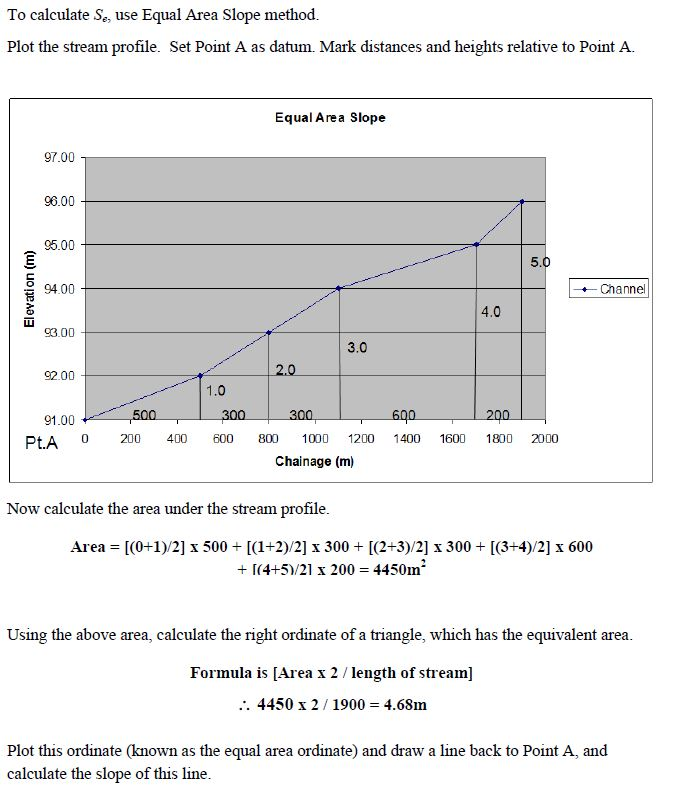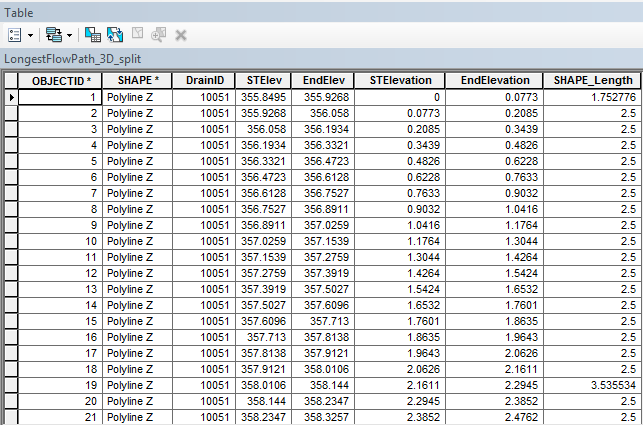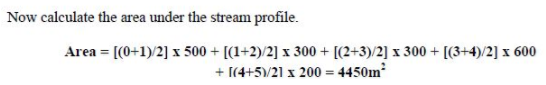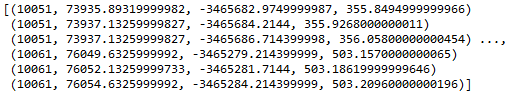# Extract Polyline Geometry Z and M - Equal Area Slope

928
2
06-21-2017 09:01 AMOccasional Contributor III

I'm currently busy writing a Python Module to determine the Equal-Area Slope of the Longest Flowpath of each catchment. I need to generate a Python Dictionary for each Longest Flowpath within the polyline Feature Class with the following format.

v = vertex

z  = Change in height (i.e. 0, 1, 2,....150)

seq = segment length between vertices (i.e. v1 - v2, 200m, v2 - v3, 300m,....v100 - v101, 500m)

longestflowpath_dict = {DrainID: [(v1(Z), v2(Z), seg1(M)),(v2(Z), v3(Z), seg2(M)),...(v100(Z), v101(Z), seq100(M))

What  approaches could I use to extract the geometry Z and M between each vertices to determine the lengths between each vertices. The Z values stored are the accumulated difference in height between each vertices.

I've included the documentation from the engineering manual with the manual methodology of determining the Equal Area Slope.Tags (3)
2 RepliesbyMVP Legendary Contributor

Peter, how are you pulling the data?  If the source is a DEM, then you can extract the values from it along a transect line using a polylline... but the trick is to draw the polyline, then densify it with a spacing approximately equal to your cell size * root 2 ( ie 10 * 1.414...  or even 10). then use the Extract to Points tool from there, you can get the X, Y values, and of course Z.  X and Y will allow you to determine distance from the start of the line (I have sequential distance between points code in my blog if you need it... field calculator expression).

Sequential distance, sequential Z an its difference with some rolling filters will provide the necessary information.

My obvious suggestion  once you get the X,Y and Z values is to send the data to numpy for further processing an graphing (ie matplotlib)  If you get a samle profile, post a copy if you want me to provide further suggestionsOccasional Contributor III

Hi Dan

Thanks for the following. In order to get the following solved before my deadline. I extracted the Z values from the DTM using the InterpolateShape_3d. I then split the polylines using SplitLine_management to split the polyline in segments. I added the the following fields: ["STElev", "EndElev", "STElevation", "EndElevation"]. I then calculated the Start Elevation and End Elevation using Calculate Geometry.Thereafter  I calculated the "STElevation" by subtracting each elevation by the initial STElev (i.e. 355.9268 - 355.8495), "EndElevation" (i.e. 355.6268 - 355.9268). I then used a Search Cursor and calculated the area for each trapezoid using the following formula:``````'''
Created on 22 Jun 2017
@author: PeterW
'''
# import modules and site packages
import arcpy

# set environment settings
arcpy.env.overwriteOutput = True

def equal_area_slope(lfp_3d, lfp_3d_split):
"""Determine the Equal Area Slope
for each Drainage Area's Longest
Flowpath"""
slope_area_dict = {}
with arcpy.da.SearchCursor(lfp_3d_split, ["DrainID", "STElevation", "EndElevation", "SHAPE@LENGTH"]) as scur: # @UndefinedVariable
for row in scur:
trap_area = ((row+row)/2)*row
slope_area_dict.setdefault(row, []).append(trap_area)
with arcpy.da.UpdateCursor(lfp_3d, ["DrainID", "EASlope", "SHAPE@LENGTH"]) as upcur: # @UndefinedVariable
for row in upcur:
if row in slope_area_dict:
slope_area = sum(slope_area_dict[row])
right_ordinate = (slope_area*2)/row
ea_slope = (right_ordinate/row)*100
row = ea_slope
upcur.updateRow(row)

if __name__ == "__main__":
lfp_3d = r"E:\Projects\2016\G113665\geoHydro\Model02\Results.gdb\LongestFlowPath_3D"
lfp_3d_split = r"E:\Projects\2016\G113665\geoHydro\Model02\Results.gdb\LongestFlowPath_3D_split"
equal_area_slope(lfp_3d, lfp_3d_split)‍‍‍‍‍‍‍‍‍‍‍‍‍‍‍‍‍‍‍‍‍‍‍‍‍‍‍‍‍‍‍‍‍‍‍‍‍‍‍‍‍‍‍‍‍‍‍‍‍‍‍‍‍‍‍‍‍‍‍‍‍‍‍‍‍‍‍‍‍‍‍‍‍‍‍‍‍‍‍‍‍‍‍‍‍‍‍‍‍‍‍‍‍‍‍‍‍‍‍‍‍‍‍‍‍‍‍‍‍‍‍‍‍‍‍‍‍‍‍‍‍‍‍‍‍‍‍‍‍‍‍‍‍‍‍‍‍‍‍‍‍‍‍‍‍‍‍‍‍‍‍‍‍‍‍‍``````

I've started re-writing the following using NumPy arrays. I've converted the 3D polyline into a NumPy array. The array contains the "DrainID" X Coordinate, Y Coordinate, Z Coordinate for each point. How do I go about replicating the following using NumPy arrays? I trying to figure out the most Pythonic way of creating an array of "DrainID", "STElevation", "EndElevation" and "SegmentLength"

Current Code:

``````''
Created on 01 Jul 2017
@author: PeterW
'''
# import modules and site-packages
import numpy as np
import pandas as pd
from pathlib import Path
import arcpy

# set environment settings
arcpy.env.overwriteOutput = True

# check out extensions
arcpy.CheckOutExtension("3D")

def longestflowpath_3d(dtm, lfp_2d):
"""Generate LongestFlowPath 3D
from LongestFlowPath 2D and DTM"""
output_gdb = str(Path(lfp_2d).parents)
lfp_3d = "{0}\\{1}".format(output_gdb, "lfp_3d")
lfp_3d = arcpy.InterpolateShape_3d(dtm, lfp_2d, lfp_3d, vertices_only=True)
arcpy.FlipLine_edit(lfp_3d)
return lfp_3d

def area_under_profile(lfp_3d):
"""Determine Area Under Profile"""
arr = arcpy.da.FeatureClassToNumPyArray(lfp_3d, ["DrainID", "SHAPE@X", "SHAPE@Y", "SHAPE@Z"], explode_to_points=True) # @UndefinedVariable
print(arr)

def equal_area_slope(dtm, lfp_2d):
"""Determine the Equal Area Slope
for each Drainage Area's Longest
Flowpath"""
lfp_3d = longestflowpath_3d(dtm, lfp_2d)
area_under_profile(lfp_3d)

if __name__ == "__main__":
dtm = r"E:\Projects\2016\G113665\geoHydro\DTM2\raw1"
lfp_2d = r"E:\Projects\2016\G113665\geoHydro\Model02\Results.gdb\LongestFlowPath_2D_Orig"
equal_area_slope(dtm, lfp_2d)‍‍‍‍‍‍‍‍‍‍‍‍‍‍‍‍‍‍‍‍‍‍‍‍‍‍‍‍‍‍‍‍‍‍‍‍‍‍‍‍‍‍‍‍‍‍‍‍‍‍‍‍‍‍‍‍‍‍‍‍‍‍‍‍‍‍‍‍‍‍‍‍‍‍‍‍‍‍‍‍‍‍‍‍‍‍‍‍‍‍‍‍‍‍‍‍‍‍‍‍‍‍‍‍‍‍‍‍‍‍‍‍‍‍‍‍‍‍‍‍‍‍‍‍‍‍‍‍‍‍‍‍‍‍‍‍‍‍‍‍‍‍‍‍‍‍‍‍‍‍‍‍‍‍‍‍‍‍‍‍‍‍‍‍‍‍‍‍‍‍‍‍‍‍‍‍‍‍‍‍‍‍‍‍‍‍‍‍‍‍‍‍‍‍‍‍‍‍‍‍‍‍‍‍‍‍‍‍‍‍‍‍‍‍‍‍‍‍‍‍‍‍‍‍‍‍‍‍‍‍‍‍‍‍‍‍‍‍‍‍‍‍‍‍‍‍‍‍‍‍‍‍‍‍‍‍‍‍‍‍‍‍‍‍‍‍‍‍‍‍‍‍‍‍‍‍‍‍‍‍‍‍‍‍‍‍‍‍‍‍‍‍‍‍‍‍‍‍‍‍‍‍‍‍‍‍‍‍‍‍‍‍‍‍‍‍‍‍‍‍‍‍‍‍‍‍‍‍‍‍‍‍‍‍‍‍‍‍``````